## La$_{30}$Ce$_{30}$Al$_{15}$Co$_{25}$金属玻璃应力松弛行为 1)

*西北工业大学力学与土木建筑学院, 西安 710072

## STRESS RELAXATION OF La$_{30}$Ce$_{30}$Al$_{15}$Co$_{25}$ METALLIC GLASS 1)

Chen Yinghong*, Wang Yunjiang, Qiao Jichao,*,2)

*School of Mechanics, Civil Engineering and Architecture, Northwestern Polytechnical University, Xi'an 710072, China

State Key Laboratory of Nonlinear Mechanics, Institute of Mechanics, Chinese Academy of Sciences, Beijing 100190, China

 基金资助: 1)国家自然科学基金.  51971178陕西省自然科学基金.  2019JM-344中央高校基本科研业务费专项资金.  3102019ghxm007中央高校基本科研业务费专项资金.  3102017JC01003西北工业大学硕士研究生创意创新种子基金.  ZZ2019014非线性力学国家重点实验室开放基金.  LNM201911

Received: 2020-01-10   Accepted: 2020-02-27   Online: 2020-05-18Abstract

Metallic glass is a well-known engineering material that has been attracting tremendous research interest in materials science and condense matter physics. Early studies of the properties and structures of the metallic glasses showed that the dynamic heterogeneity is closely linked to the viscoelasticity and plasticity of metallic glasses. However, the physical landscape between the macroscopic stress relaxation behavior and the mechanical relaxation is still obscure. Different from the deformation mechanism of their crystalline counterparts, the deformation mechanism of metallic glasses is more complicated. To fully understand the mechanical properties of metallic glass, it is necessary to ascertain the structural characteristics of different spatial scales of metallic glass and evolution of structural characteristics with time. The significant importance is the connection between the macroscopic stress relaxation behavior and the dynamic mechanical relaxations ($\beta$ relaxation, or $\alpha$ relaxation) in metallic glasses. Stress relaxation is a robust technique to characterize the viscoelastic and plastic mechanisms in glasses which can reflect their structural and dynamic heterogeneities. In the current research, La$_{30}$Ce$_{30}$Al$_{15}$Co$_{25}$ metallic glass was used as a model system, dynamic mechanical processes and stress relaxation behavior were studied. Compared with other traditional metallic glasses, La$_{30}$Ce$_{30}$Al$_{15}$Co$_{25}$ metallic glass shows a pronounced $\beta$ relaxation process. The analysis based on the Kohlarausch-Willams-Watts (KWW) equation suggests that the stress relaxation process of metallic glass is a heterogeneous dynamic process. We observed an unusual two-stage stress relaxation phenomenon, consisting of the fast stress-driven event and the slow thermally activated event. The two-stage stress relaxation behavior is attributed the stress-driven event and thermally activated event to short-range atomic rearrangement, and long-range atomic diffusion, respectively. In addition, the analysis of the activation energy spectrum shows that the activation of the stress relaxation unit is not uniform, which corresponds to fluctuations in energy. This research is a step towards building a bridge linking the structural and dynamic heterogeneity of metallic glasses, and strongly supports the physical scenario of $\beta$ to $\alpha$ relaxation.

Keywords： metallic glass ; dynamic relaxation ; stress relaxation ; structural heterogeneity ; activation energy spectrum model

Chen Yinghong, Wang Yunjiang, Qiao Jichao. STRESS RELAXATION OF La$_{30}$Ce$_{30}$Al$_{15}$Co$_{25}$ METALLIC GLASS 1). Chinese Journal of Theoretical and Applied Mechanics[J], 2020, 52(3): 740-748 DOI:10.6052/0459-1879-20-013

## 2 实验结果与讨论

### 图1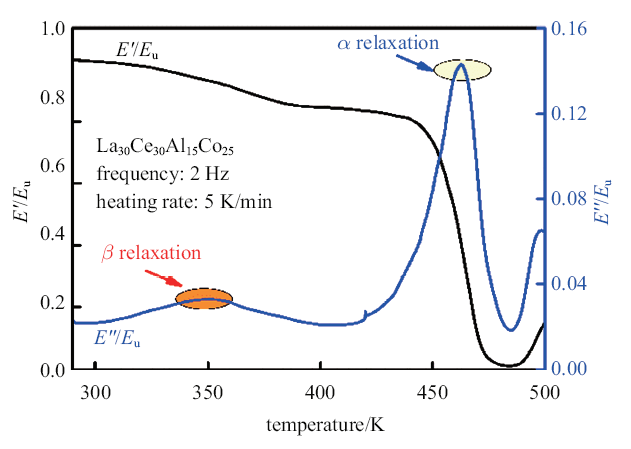Fig.1   Normalized loss modulus and storage modulus of La$_{30}$Ce$_{30}$Al$_{15}$Co$_{25}$ metallic glass as a function of temperature. $E_{u}$ assumes the storage modulus at room temperature

### 图2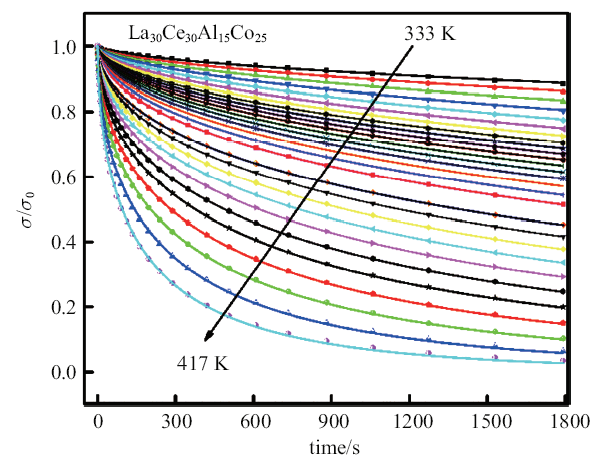Fig.2   Stress relaxation spectra of La$_{30}$Ce$_{30}$Al$_{15}$Co$_{25}$ metallic glass at different temperatures (333$\sim$417 K). The solid lines are the KWW fittings

$\sigma =\sigma _0 \exp \lt[-\lt(\frac{t}{\tau })^{\beta _{KWW}}]$

### 图3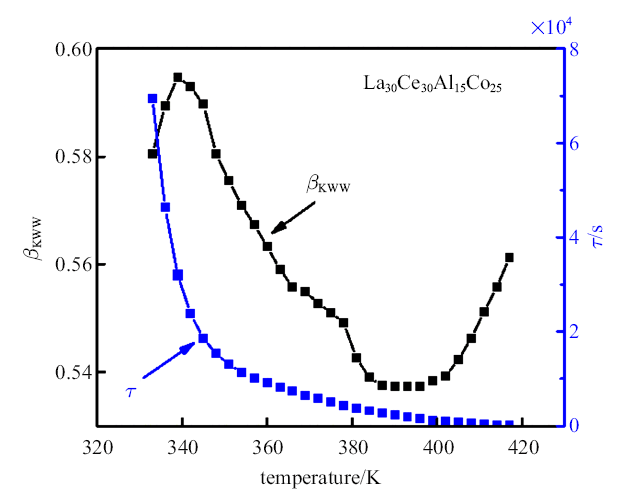Fig.3   The KWW fitting parameters $\beta_{KWW}$ and $\tau$ of La$_{30}$Ce$_{30}$Al$_{15}$Co$_{25}$ metallic glass as a function of temperature

### 图4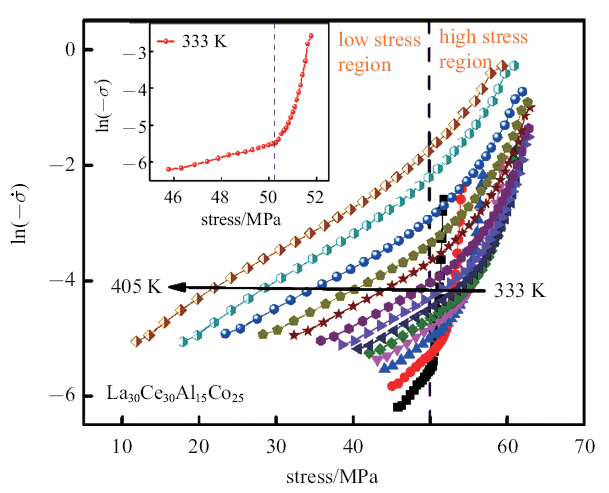Fig.4   $\ln (\dot{\sigma})$ of La$_{30}$Ce$_{30}$Al$_{15}$Co$_{25}$ metallic glass as a function of stress. The inset shows an example at a given temperature of 333~K, which shows an obvious change of stress relaxation with the variation of stress

$\varepsilon (t)=\frac{\sigma (t)}{E}+\varepsilon_{p} (t)$

$\dot{\varepsilon}(t)=\frac{\dot{\sigma}(t)}{E}+\dot{\varepsilon}_{p} (t)$

$\frac{\dot{\sigma}}{E} =-\dot{\varepsilon}_{p}$

$\dot{\varepsilon}_{p}=\dot{\varepsilon}_0 \exp \lt(\frac{\Delta Q-\dfrac{\sigma }{3}\varOmega }{K_{B} T})$

$\varOmega =\sqrt 3K_{B} T\frac{\partial \ln (-\dot{\sigma})}{\partial \sigma }$

$\Delta H(\sigma )=-\frac{\partial \ln (-\dot{\sigma})}{\partial [1/(K_{B} T)]}$

### 图5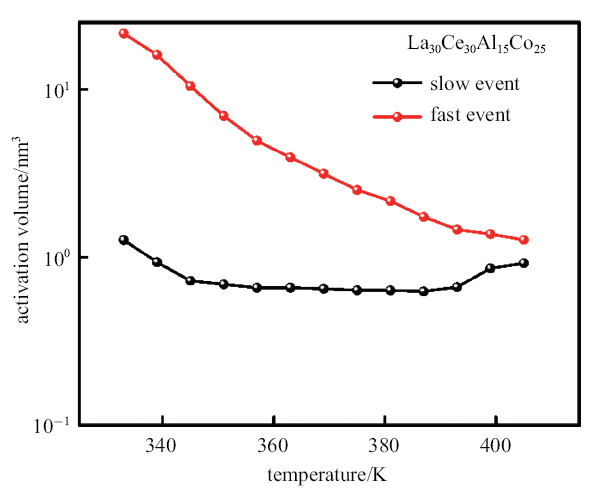Fig.5   Evolution of the derived activation volume of fast and slow process in La$_{30}$Ce$_{30}$Al$_{15}$Co$_{25}$ metallic glass with temperature

### 图6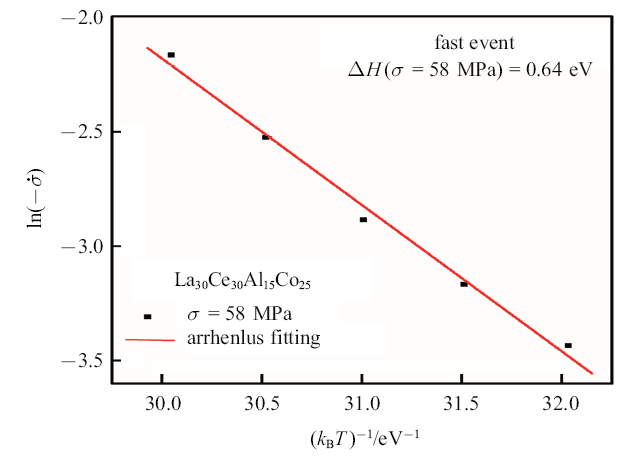Fig.6   Activation enthalpy of La$_{30}$Ce$_{30}$Al$_{15}$Co$_{25}$ metallic glass with a characteristic stress at 58~MPa for fast event

### 图7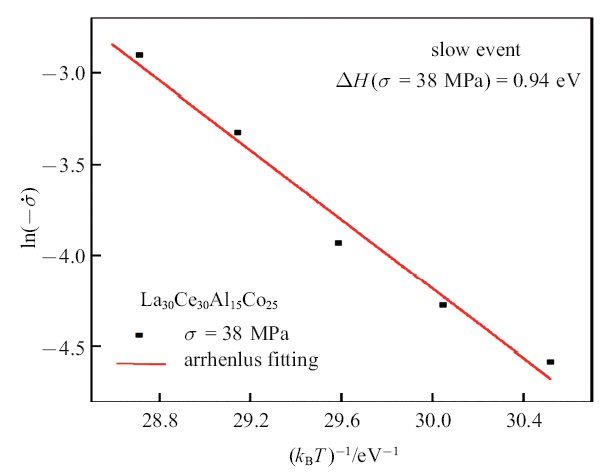Fig.7   Activation enthalpy of La$_{30}$Ce$_{30}$Al$_{15}$Co$_{25}$ metallic glass with a characteristic stress at 38 MPa for slow event

$\Delta \sigma (t)=\int_0^{+\infty} P(E)\theta (E, T, t){d}E$

$\theta (E, T, t)=1-\exp \lt[-v_0 t\exp \lt(-\frac{E}{K_{B} T})]$

$P(E)=-\frac{1}{K_{B} T}\frac{{d}\sigma (t)}{{d}\ln (t)}$

$E=K_{B} T\ln (v_0 t)$

### 图8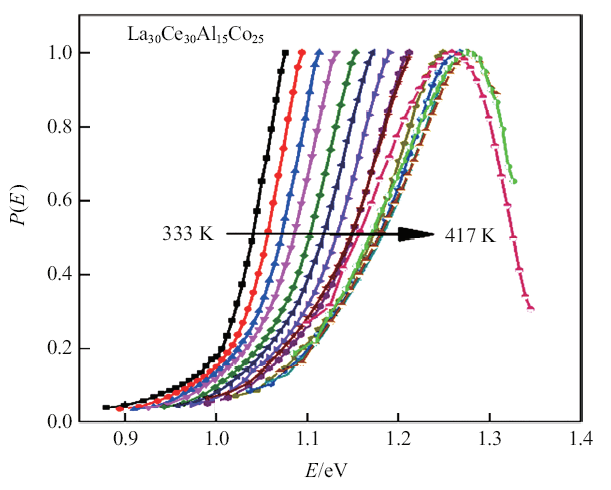Fig.8   Evolution of activation energy spectrum with temperature in La$_{30}$Ce$_{30}$Al$_{15}$Co$_{25}$ metallic glass

## 3 结论

(1) La$_{30}$Ce$_{30}$Al$_{15}$Co$_{25}$金属玻璃具有明显的慢$\beta$弛豫行为.

(2) La$_{30}$Ce$_{30}$Al$_{15}$Co$_{25}$金属玻璃金属玻璃化应力松弛呈现双阶段现象, 存在由应力驱动的快过程向热激活的慢过程转变, 基于一种热动力学理论分析这两个阶段介于金属玻璃动态弛豫中的弛豫和$\beta$弛豫之间.

(3) 对松弛数据基于激活能谱模型的分析发现, 松弛单元的激活并不是均一化的, 存在着能垒的变化, 从能量角度反映了金属玻璃的动力学和结构非均匀性.

/

 〈〉